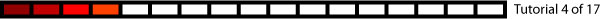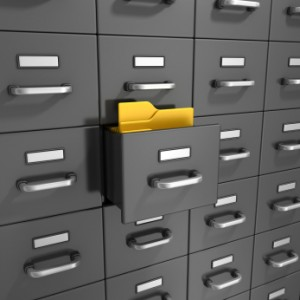# Data inside PLCsThink of PLC memory as a cabinet with drawers called Registers.  Data is held in these Registers.These Registers come in different sizes, and hold different kinds of data.

Bits: Can have values 1 or 0.  1 typically represents On while 0 represents Off.  Bits are the building block for all other types of data.
Integers:  Whole numbers (no decimal points).  Called: Characters (char), Integers (int), long Integers (long int) or Words.  Look for the bit size and whether they are signed or unsigned.   Unsigned are positive numbers, while signed are positive or negative.
Floating point numbers
: Numbers with decimal points, and can be positive or negative.They are called floating point numbers (Float), with their larger variety called double floats.

Type# bitsSigned/UnsignedMin ValueMax Value
Bit101
Int8Signed-128127
Unsigned0255
16Signed-32,76832,767
Unsigned065,535
32Signed-2,147,483,6482,147,483,647
Unsigned04,294,967,295
Floating Point321.175 E–383.403 E+38
642.225 E–3081.798 E+308

Diving Deeper

Inside the Data Types: As stated earlier, all Data Types are made of Bits (1’s and 0’s). An 8 bit number is written like bbbbbbbb (where “b” can represent a 0 or 1),  so you may have 00000000, 11111111 or 01011110…. any combo will do.  What these Bits mean is determined by the Data Type.

Unsigned Integers: The least valuable bit is the rightmost bit and double in value each position you move left.  The right-most bit is 1 it’s worth 1  next bit to the left 2   4   8  16   32  64 128 256  …… (this goes on for as many bits as the Data Type calls for).  Here’s what is looks like for an Unsigned 8-bit Integer:

If all 8 bits are 0 (00000000) then we get 0 + 0 + 0 + 0 + 0 + 0 + 0 + 0 = 0
If all 8 bits are 1 (11111111) then we get 128 + 64 + 32 + 16 + 8 + 4 + 2 + 1 = 255
So the range of an 8 bit unsigned Integer is 0 – 255
An example of something in between (00110101)  0 + 0 +  32 + 16 + 0 + 4 + 0 + 1 = 53

Signed Integers:  They’re a tad more complicated check out wikipedia’s article on 2’s compliment for a good explination.

Hexadecimal Format (Hex):  Instead of writing every bit out, it is common to group sets of 4 bits together. Each group can have a value of 0 – 15, which causes a problem since our number system goes from 0 – 9, so we also use A, B, C, D, E and F to get a total of 16 values. (0, 1, 2, 3, 4, 5, 6, 7, 8, 9 , A, B, C, D, E, F)

Example 1001 0011 1111 0010 in Hex would be 93F2

To be clear that we’re using Hex format we tack on a ‘0x’ the the start of the number so our last example would be written 0x93F2

Characters: (typically 8 bit unsigned integers) are often used to reprent letter or symbols.   Example: you may use them to display text on an operator panel. Use an ASCII table to see how values are mapped to symbols.

What about the bits in Floating Point numbers, how do they break out? It get’s a bit complicated, I don’t really want to get into that in this tutorial.   It is unlikely as a PLC user that you will ever need to know how their bits translate to values, so don’t worry about it.